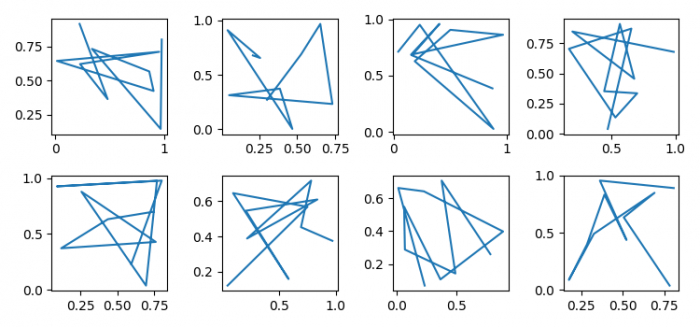# How to unset 'sharex' or 'sharey' from two axes in Matplotlib?

To inset sharex and sharey from two axes in matplotlib, we can use 'none', i.e., False or 'none'. Each subplot X- or Y-axis will be independent.

## Steps

• Set the figure size and adjust the padding between and around the subplots.

• Initialize two variables rows and cols.

• Create a figure and a set of subplots.

• Iterate the axes where rows=2 and cols=4.

• Plot the random data on the axis.

• To display the figure, use show() method.

## Example

import matplotlib.pyplot as plt
import numpy as np

plt.rcParams["figure.figsize"] = [7.50, 3.50]
plt.rcParams["figure.autolayout"] = True

rows = 2
cols = 4

fig, axes = plt.subplots(rows, cols, sharex='none', sharey='none', squeeze=False)

for row in range(rows):
for col in range(cols):
axes[row][col].plot(np.random.rand(10), np.random.rand(10))

plt.show()

## Output

It will produce the following output −